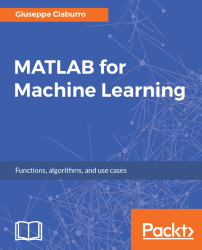•#### MATLAB for Machine Learning#### Overview of this book

MATLAB is the language of choice for many researchers and mathematics experts for machine learning. This book will help you build a foundation in machine learning using MATLAB for beginners. You’ll start by getting your system ready with t he MATLAB environment for machine learning and you’ll see how to easily interact with the Matlab workspace. We’ll then move on to data cleansing, mining and analyzing various data types in machine learning and you’ll see how to display data values on a plot. Next, you’ll get to know about the different types of regression techniques and how to apply them to your data using the MATLAB functions. You’ll understand the basic concepts of neural networks and perform data fitting, pattern recognition, and clustering analysis. Finally, you’ll explore feature selection and extraction techniques for dimensionality reduction for performance improvement. At the end of the book, you will learn to put it all together into real-world cases covering major machine learning algorithms and be comfortable in performing machine learning with MATLAB.
Title PageCreditsForewordwww.PacktPub.comCustomer FeedbackPrefaceFree Chapter
Getting Started with MATLAB Machine LearningImporting and Organizing Data in MATLABFrom Data to Knowledge DiscoveryFinding Relationships between Variables - Regression TechniquesPattern Recognition through Classification AlgorithmsIdentifying Groups of Data Using Clustering MethodsSimulation of Human Thinking - Artificial Neural NetworksImproving the Performance of the Machine Learning Model - Dimensionality ReductionMachine Learning in Practice## Regression Learner App

Previously, we described some MATLAB apps. They make fast and easy what is long and laborious. Some procedures that require the use of many functions are automated by a user-friendly environment. Additionally, we do not have to remember the names of all the functions useful to perform a specific analysis, as the graphical interface provides us with all the features available.

The Regression Learner App leads us into a step-by-step regression analysis. Through this app, import and explore data, select features, specify validation schemes, train models, and evaluate results, will be extremely simple and fast.

We can run automated training to look for the best regression model type, including linear regression models, regression trees, Gauss process regression models, vector support vehicles, and regression tree complexes. To reuse the model with new data or to perform a programmatic regression, we can export the model to the workspace or generate the MATLAB code to recreate...